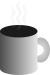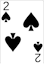#Coffee SpaceListen:

## Upper Bound Computation

TL;DR: Just a little realisation I had whilst working on a problem and I thought I would share for the benefit of others.

I’ve been working on a side project for a while now which involves a very long simulation. I won’t go into this now as it will be a later article on perhaps even paper, but to say the least, it’s a really long running computational problem.

Anyway, I had a simple question to answer: Given some arbitrary computation that may or may not halt, how long should it be left so that all possible computation is complete?

As is quite well known, it is not possible to determine whether some arbitrary problem will ever halt. The next best thing we can do is to run it long enough such that if it is possible to halt, then it does.

### Example

One example problem I had was Langton’s Ant. Technically Langton’s Ant is Turing complete as it is capable of doing arbitrary computations. It may or may not return to its original state.

We consider a version of the problem where the sides of the world wrap around, otherwise we know the agent is capable of creating infinite “highways”.

We have a world of patches with length $l$, for a total number of $l^2$ patches. Each patch $p$ has a state of $\{ 0, 1 \}$ (representing black or white). This gives $2^{l^2}$ states of the world.

The agent $R$ can be in any $(x,y)$ location, for a total of $l^2$ unique locations. The agent can also be in a direction $\alpha$ of $\{ NORTH, EAST, SOUTH, WEST \}$ ($|\alpha| = 4$). This gives $l^2 \times |\alpha|$ states of the agent.

For some arbitrary size world, we therefore have $2^{l^2} \times l^2 \times |\alpha|$ total states of the world.

### Generalization

It occurred to me that this is more general still. Here we make the following assumptions:

1. Program memory and dynamic memory is shared and calculated.
2. Nothing is done outside of this memory (we do not consider registers or cache for simplicity).
3. We are able to calculate the length of this space used.

This means that given the length of some memory (assuming in bits) $|M|$, we know that if a program will exit, it most definitely will happen in less than $2^{|M|}$.

Of course, for large functions, this state space is enormous, perhaps even possible to check. But, we actually do know the difference between ‘data’ and ‘code’ sections, so we can lessen this search space still. Therefore for small functions, we could define a reasonable upper bound for when a function must end.

For example, consider the following arbitrary example:

0001 uint8_t foo(uint8_t a, uint8_t b, uint8_t c){
0002   uint8_t z = 0;
0003   while(a + b + z != c){
0004     some_process(a, b);
0005     ++z;
0006     if(z >= 128) z = 0;
0007   }
0008   return z;
0009 }

We have three input states, $a$, $b$, $c$, for a total number of bits $2^{8 + 8 + 8}$. These are used to seed the environment, meaning we will need to do this number of tests to completely test the problem.

The question is now, how long do we wait? Well, as our active internal data consists of just $z$, we know that we have explored all possible states after $2^8$ loops. If a solution is not found then, we can assume that there is not a solution.

A solution may not exist for example when $a + b + 128 \leq c$, and would result in an infinite loop. Of course for testing, this is no good. We could have a watchdog that checks for long running processes, but how long do we wait? What if some_process() takes a while to run?

Anyway, I thought I would share this. Hopefully you find it as interesting as I do.